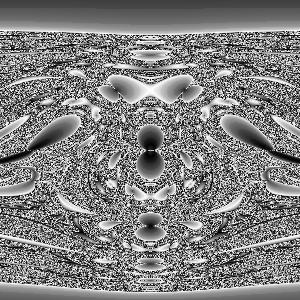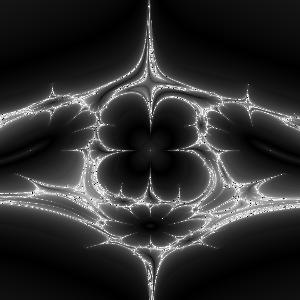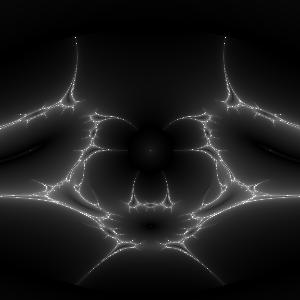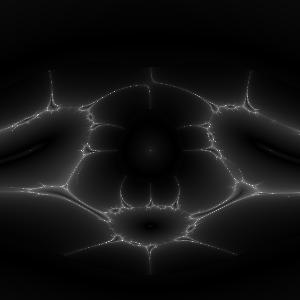Theory.org » fracdyn » whirlpool » The Whirlpool: Graph

 n = 1 n = 2 n = 3 n = 4 k = 1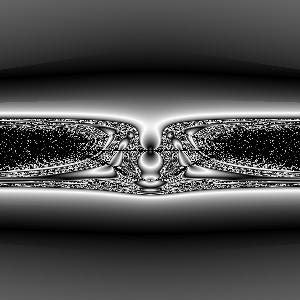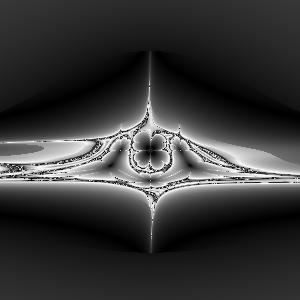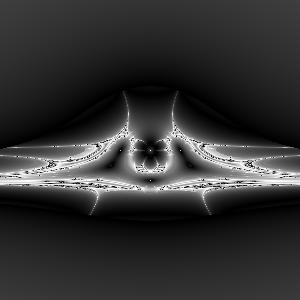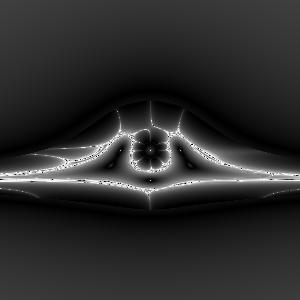k = 2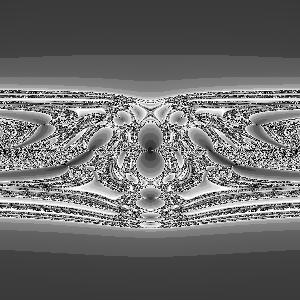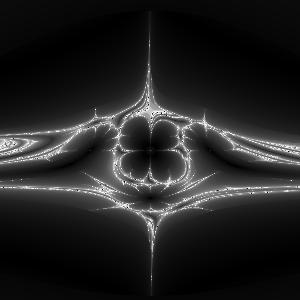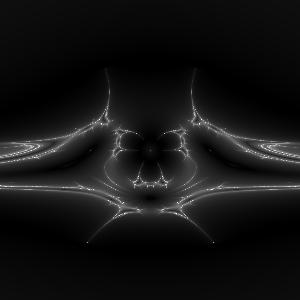k = 3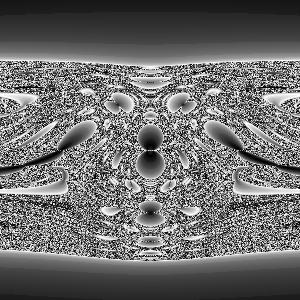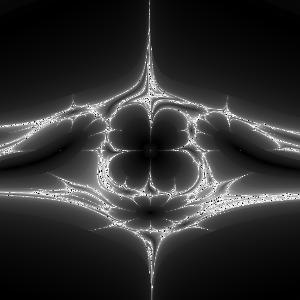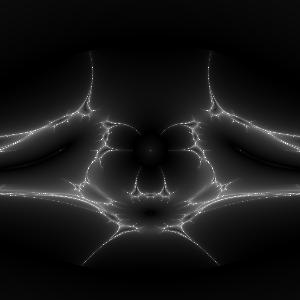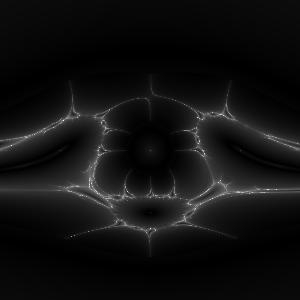k = 4